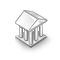Interesting script?
So post a link to it -
let others appraise it
You liked the script? Try it in the MetaTrader 5 terminal# Maximum Percentage of Equity Risk - library for MetaTrader 5

Views:
4771
Rating:
Published:
2014.08.01 10:35
Updated:
2016.11.22 07:32

This code allows you to set a maximum percentage of equity risk.

It checks if asked buy/sell lots are fitting the risk. If not, lots are automatically adjusted to fit the risk that was set.

Code:

```bool UseMaximumPercentageRisk=true;
double MaximumPercentageRisk=25;

//+------------------------------------------------------------------+
//+------------------------------------------------------------------+
double GetLotSize(double lotsize)
{
//--- Gets pair specs
CSymbolInfo symInfo;
int  digits_bn=symInfo.Digits();
double  points_bn=symInfo.Point();
string symbol_bn=_Symbol;
int tmpdecimal=1;
double old_lot=lotsize;
//---
if((NormalizeDouble(AccountInfoDouble(ACCOUNT_FREEMARGIN)*(MaximumPercentageRisk/100)/1000.0,tmpdecimal)<lotsize) && UseMaximumPercentageRisk) //is lot fitting risk ?
{
lotsize=NormalizeDouble(AccountInfoDouble(ACCOUNT_FREEMARGIN)*(MaximumPercentageRisk/100)/1000.0,tmpdecimal);  //Calculates new Lotsize

if(lotsize<SymbolInfoDouble(symbol_bn,SYMBOL_VOLUME_MIN)) //is LotSize fitting minimum broker LotSize ?
{
lotsize=SymbolInfoDouble(symbol_bn,SYMBOL_VOLUME_MIN);   //No! Setting LotSize to minimum's broker LS
Print(_Symbol," Lot adjusted from ",old_lot," to minimum size allowed by the server of ",lotsize);
}
else
{
Print(_Symbol," Lot adjusted from ",old_lot," to ",lotsize," to comply with Maximum Risk condition. Each trade can risk only ",MaximumPercentageRisk,"% of free margin.");   //Yes!
if(MathAbs(lotsize/SymbolInfoDouble(symbol_bn,SYMBOL_VOLUME_STEP)-MathRound(lotsize/SymbolInfoDouble(symbol_bn,SYMBOL_VOLUME_STEP)))>1.0E-10) //Is LotSize fitting Broker's allowed step ?
{
lotsize=SymbolInfoDouble(symbol_bn,SYMBOL_VOLUME_STEP)*NormalizeDouble(lotsize/SymbolInfoDouble(symbol_bn,SYMBOL_VOLUME_STEP),0);   //NO! recalculates LotSize.
Print("M-",_Symbol," Warning: Your calculated percentage at risk lot size of was not a multiple of minimal step",SymbolInfoDouble(symbol_bn,SYMBOL_VOLUME_STEP),". Lot size changed to",lotsize);
}
}
}
return(lotsize);
}```FxTrend 25EMA

FxTrend 25EMA is based on the difference of the EMA 25 value during two different moments.Astro Indicators

Show the aspect of two planets, the declination of planets or just the Body position.Mercado Aberto

Simple indicator that shows when market is open.CoensioTrader1V06

A multi-currency trading system, based on Bollinger Bands and trend catching technique. Capable of trading up to 6 currencies simultaneously. System has build in optimization parameter sharing.